• 最近在做计算方法大作业，其中在三次样条插值的算法实现中，输入列表x_i直接复制x，但之后出现奇异现象：函数中没有对x_i的改变，为什么x_i无缘无故更改呢？ 原来，python中的列表是可变对象，直接赋值操作不会...
记录一下Python列表赋值踩过的坑
最近在做计算方法大作业，其中在三次样条插值的算法实现中，输入列表x_i直接复制给了x，但之后出现奇异现象：函数中没有对x_i的改变，为什么x_i无缘无故更改呢？
原来，python中的列表是可变对象，直接赋值操作不会分配新内存来存储变量！
注意区分以下不同：
1.列表赋值
2.列表浅拷贝
3.列表深拷贝


展开全文• 微信小程序，使用 setData 两个列表变量赋值同一个数据时，当其中一个列表变量发生改变，另外一个变量也会随之改变，例如 wx.request({ url: app.url + 'api/getSelect', header: { 'Content-Type': '...
微信小程序，使用 setData 给两个列表变量赋值同一个数据时，当其中一个列表变量发生改变，另外一个变量也会随之改变，例如

wx.request({
url: app.url + 'api/getSelect',
'Content-Type': 'application/x-www-form-urlencode;charset=utf-8',
'token': app.globalData.token
},
success: function (res) {
if (res.data.code == 0) {
that.setData({
})
} else {
wx.showToast({
title: res.data.msg,
icon: 'none',
duration: 2000
})
setTimeout(function () {

wx.navigateBack({
delta: 1,
})
}, 2000);
}
}
});

这是因为 之类的赋值  js 仅仅做了浅拷贝，只是赋值了引用，所以在改变一个列表变量的时候改变的是元数据，而

这两个指向元数据的变量 就会同时改变

解决办法：

使用 json.stringify  和 json.parse 重新赋值一个新的 变量list


wx.request({
url: app.url + 'api/getSelect',
'Content-Type': 'application/x-www-form-urlencode;charset=utf-8',
'token': app.globalData.token
},
success: function (res) {
if (res.data.code == 0) {
that.setData({
})
} else {
wx.showToast({
title: res.data.msg,
icon: 'none',
duration: 2000
})
setTimeout(function () {

wx.navigateBack({
delta: 1,
})
}, 2000);
}
}
});


展开全文javascript
• 我有这个：rows = [[convert[random.randint(0,7)] for _ in range(5)] for _ in range(5)]printedrows = [("[X]","[X]","[X]","[X]","[X]"," 这将创建5行随机值：^{pr2}$我想把“X”重新分配数字，这样它的读数... 当前要分配我的变量，我有这个：rows = [[convert[random.randint(0,7)] for _ in range(5)] for _ in range(5)]printedrows = [("[X]","[X]","[X]","[X]","[X]"," 这将创建5行随机值：^{pr2}$我想把“X”重新分配给数字，这样它的读数如下：('[X]', '', '[X]', '[X]', '[X]', '  ('', '[X]', '', '', '', '  ('[X]', '[X]', '', '[X]', '[X]', '  ('', '[X]', '[X]', '[X]', '', '  ('[X]', '', '[X]', '', '[X]', '  但是，当我使用这个时：(仅示例-位置由用户决定)convert = {0:0,1:1,2:2,3:3,4:0,5:1,6:2,7:1}printedrows[y][x] = rows[y][x]TypeError: 'tuple' object does not support item assignment我能做些什么来解决这个问题，这样我就可以在没有错误的情况下更改值了吗？谢谢。在
展开全文• for x in range(columns): for y in range(rows): if (BW[y][x] == 255): phi = ang[y][x] i = round((phi + (math.pi / 2)) / d) #print(i) if (i == 0): i = 1 v = F[i] + 1 if (v > s): s =...python
• import numpy as np ...#tp=[0 for x in range(10)] tp=[] import matplotlib.pyplot as plt k=0 for i in range(10): # print(reward_his[i]) if (i > 2) and (i % 2 == 0): print(reward_his[i]) tp[k]=r
直接来正确的代码
我的目的是“从一个列表中抽取几个元素（index大于2且为偶数）赋值给我的新的列表”
"""
Created on Sun Jun 28 17:18:32 2020

@author: han
"""
import numpy as np

reward_his=np.random.randint(1,10,10) #一个cpu周期的本地的能耗

tp=[0 for x in range(10)]

import matplotlib.pyplot as plt
k=0
for i in range(10):
#   print(reward_his[i])
if (i > 2) and (i % 2 == 0):##脉冲可能就ius因为她
print(reward_his[i])
tp[k]=reward_his[i]
#        tp[k].append(reward_his)
k+=1

a=tp[0:k]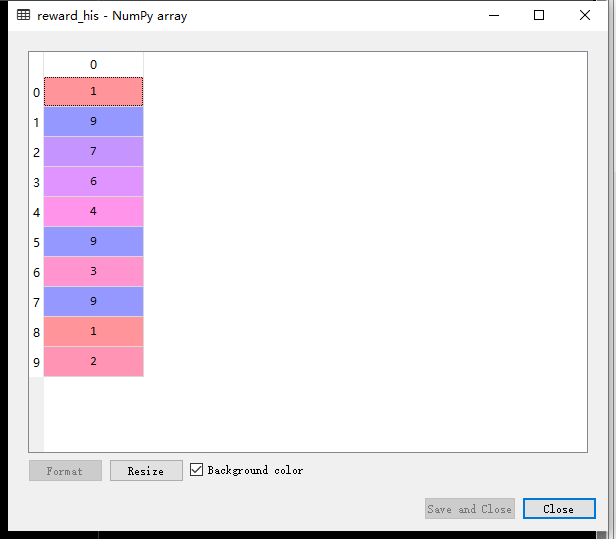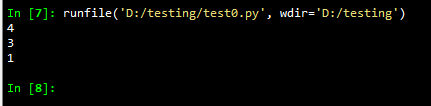接下来是错误的代码
import numpy as np

reward_his=np.random.randint(1,10,10)

#tp=[0 for x in range(10)]
tp=[]
import matplotlib.pyplot as plt
k=0
for i in range(10):
#   print(reward_his[i])
if (i > 2) and (i % 2 == 0):
print(reward_his[i])
tp[k]=reward_his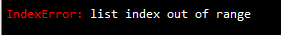小编我昨天彭搭配这么一个问题
然后将列表改为append加入元素也不对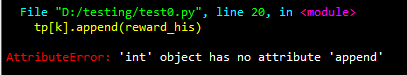正确的代码为
"""
Created on Sun Jun 28 17:18:32 2020

@author: han
"""
import numpy as np

reward_his=np.random.randint(1,10,10) #一个cpu周期的本地的能耗

tp=[0 for x in range(10)]
import matplotlib.pyplot as plt
k=0
for i in range(10):
#   print(reward_his[i])
if (i > 2) and (i % 2 == 0):##脉冲可能就ius因为她
print(reward_his[i])
tp[k]=reward_his

这段代码也有问题
  tp[k]=reward_his

这一步右边的列表没加序号导致结果如下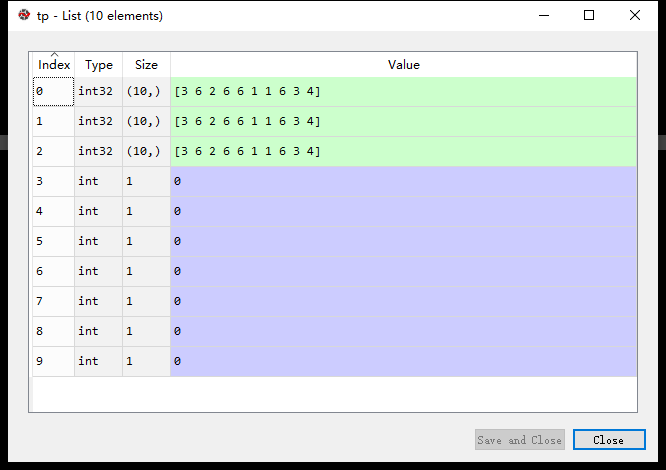展开全文• //查看对象的引用计数增加对象的引用计数场景对象创建时：以赋值的方式，创建变量名的同时就会创建变量将对象添加进容器时：类似list.append()当对象被当作参数传递函数时多重目标赋值时：s1 = s2 = s3 = ‘abc...
• 文章目录描述赋值浅拷贝通过切片拷贝通过list()函数复制通过列表extend()方法复制通过copy模块...一般来说，对于普通变量x,y，直接把x值赋y就能让两个变量相同 >>> x = 1 >>> id(x) 2093991856...python
• 1.解压序列赋值给多个变量 方案：变量和序列中的值要相等 注意序列，可迭代对象 包括:列表、元祖、字符串、文件、迭代器和生成器 x=[1,2,3,4] # 变量和序列中的值要相等 a,b,c,d=x print(a,b,c,d) #1 2 3 4 2....python
• #函数是可以赋值给变量的 # def add(a,b): # return a + b # x = add # print(x(1,2)) #意义何在？#写一个函数，给列表中的数字【排序】 def bigger(s1,s2): return s1 > s2 def smaller(s1,s2): return s1 &...
• 初始化列表不等同于函数体内赋值，先通过初始化列表给初始值，然后再执行函数体内的操作。 如果没有显示指明初始化列表，就会先按照默认值初始化一遍，再执行函数体内的操作。把赋值操作放在函数体内，相当于初始化...c++
• 在给多个变量赋值时，我们时常用“ a, b, c = 1, 2, 3”这种赋值方式，但是当我们需要将列表、元组等可迭代对象(iterable)中的元素分别赋值给多个变量时，又该如何做呢？ 方法一：通过简单的赋值操作将序列分解 >...
• 列表赋值：如果成员为const类型或者是引用类型（&）则可以使用该方法成员赋初值。 // 类列表初始化 class Person { public: // 直接复制传成员 Person(int _x, int _y); // 列表方式 Person(int _x, int...c++
• 这种赋值可以用于任何可迭代对象，包括：列表、元祖、字符串、迭代器、生成器等。 当只需要解压一部分数据时，可以通过任意变量名的方式占位。 如： >>> data = [ 'ACME', 50, 91.1, (2012, 12, 21) ] ...
• 1.解压序列赋值给多个变量 只要变量的数量和元素的个数相同就可以如此简单的解压赋值 p = (4,5) x,y=p 实际上这种解压赋值方法可以用在任何可迭代对象上面（） 转载于:...
• Lua 可以对多个变量同时赋值，变量列表和值列表的各个元素用逗号分开，赋值语 句右边的值会依次赋左边的变量 a, b = 10, 2*x <--> a=10; b=2*x 遇到赋值语句 Lua 会先计算右边所有的值然后再执行赋值操作...
• X-axis of point F",fontsize=14) plt.ylabel("Y-axis of point F",fontsize=14) plt.scatter(xFs,yFs,c='blue',edgecolor='none') plt.show()#布置画布并输出轨迹图 ...python 有问必答
• 赋值语句你见过很多，有的变量赋值，还有的数据结构的一部分（如列表中的元素和切片，或者字典项）赋值，但还有其他类型的赋值语句。例如，可同时（并行）多个变量赋值： >>> x, y, z = 1, 2, 3 ...
• 函数(1)数学定义：y=f(x),y是x的函数，x是自变量(2)python函数：由若干语句组成的语句块，函数名称，参数列表构成，它是组织代码的最小单元,完成一定的功能。可以通过函数名在程序的不同地方多次执行(这通常叫做函数...
• iamlaosong文曾经看到这样一个问题，一个字典中的元素是列表，将这个列表元素赋值给一个变量，然后修改这个列表中元素的值，结果发现，字典中那个列表也同样修改了。那个问题如下：dict = {'a':[1,2,3,4,5],'b':2}x ...
• 曾经看到这样一个问题，一个字典中的元素是列表，将这个列表元素赋值给一个变量，然后修改这个列表中元素的值，结果发现，字典中那个列表也同样修改了。那个问题如下：dict = {'a':[1,2,3,4,5],'b':2}x = dict['a']...
• Lua可以对多个变量同时赋值，变量列表和值列表的各个元素用逗号分开，赋值语句右边的值会依次赋左边的变量。 a, b = 10, 2*x <--> a=10; b=2*x 遇到赋值语句Lua会先计算右边所有...
• ## LUA教程赋值语句-13

千次阅读 2020-07-12 09:43:57
Lua像C和PASCAL几乎支持...Lua可以对多个变量同时赋值，变量列表和值列表的各个元素用逗号分开，赋值语句右边的值会依次赋左边的变量。 a, b = 10, 2*x <--> a=10; b=2*x 遇到赋值语句Lua会先计算右边所有.lua lua脚本语言
• 曾经看到这样一个问题，一个字典中的元素是列表，将这个列表元素赋值给一个变量，然后修改这个列表中元素的值，结果发现，字典中那个列表也同样修改了。 那个问题如下： dict = {'a':[1,2,3,4,5],'b':2} x = dict['...
• 赋值语句 注释，用(--)来表示 定义，lua中没有定义...Lua可以对多个变量同时赋值，变量列表和值列表的各个元素用逗号分开，赋值语句右边的值会依次赋左边的变量。a, b = 10, 2*x a=10; b=2*x  遇到赋值语句
• Lua可以对多个变量同时赋值，变量列表和值列表的各个元素用逗号分开，赋值语句右边的值会依次赋左边的变量。 a, b = 10, 2*x <--> a=10; b=2*x 遇到赋值语句Lua会先计算右边所有的值...
• python 的解压赋值可以用在任何可迭代对象上面，而不仅仅是列表或者元组。 包括字符串，文件对象，迭代器和生成器 In : s='cxk' In : a,b,c=s In : a Out: 'c' In : b Out: 'x' In : c ...
• 赋值语句见过很多，有的变量赋值，还有数据结构的一部分（如列表中的元素和切片、或字典项）赋值，但还有其它类型的赋值。 同时（并行）多个变量赋值： >>> x, y, z = 1, 2, 3 >>> ...
• 赋值是改变一个变量的值和改变表域的... Lua 可以对多个变量同时赋值，变量列表和值列表的各个元素用逗号分开，赋值语 句右边的值会依次赋左边的变量。 a, b = 10, 2*x &lt;--&gt; a=10; b=2*x  遇到...lua...